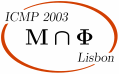ICMP 2003 > Sessions > Path integrals and stochastic analysis

# Path integrals and stochastic analysis

Session organized by S. Albeverio (Bonn), G. Ben Arous (Lausanne).

## Talks

 R. Leandre Nancy T. Levy Strasbourg S. Mazzuchi Trento H. Owhadi Marseille M. Roeckner Bielefeld A. Sengupta Baton Rouge

Remi Leandre
Nancy

Connes-Hida Calculus and index theory

The goal of this talk is to mix the tools of non-commutative differential geometry and Hida Calculus. In a first part, we define a Feynman path integral on a manifold as an Hida distribution, Wiener chaos being replaced by Chen iterated integrals. In a second part, we study Witten current as an Hida distribution, in order to study the contribution of the auxiliary bundle in the Index theorem and the role of the Bismut-Chern character. In a third part, we show that the J.L.O. cocycle for the Index theorem for families is an Hida distribution.

Thierry Levy
Strasbourg

Wilson loops vs spin networks

In lattice gauge theory, Wilson loops are commonly used as observables. However, spin networks are, from a mathematical point of view, much more natural. In particular, at least when the structure group is compact, it is easy to prove that spin networks separate the points on the configuration space. Extending a result of A. Sengupta, we prove the same property for the set of Wilson loops when the structure group is a finite product of orthogonal, unitary and symplectic groups.

Sonia Mazzuchi
Trento

Some new developments of Feynman path integrals and applications

A survey of recent developements concerning rigorously defined infinite dimensional oscillatory integrals, "Feynman path integrals", is given. Both the theory and its applications in quantum theory are discussed. As for the theory, general results are discussed including the case of polynomially growing phase functions, which are handled by exploiting the connection with probabilistic functional integrals. Applications to continuous measurement theory and stochastic Schrödinger equation are also discussed.

Marseille

Averaging versus chaos in turbulent transport?

The turbulence phenomenon has been addressed through two main theoretical axes. Along the first one, the flow is considered as a random vector field and averaging (space, time, ensemble) is employed to obtain statistical order (Reynolds, Richardson and Kolmogorov). With the second one the flow is assumed to be stationary and deterministic, then its evolution is obtained by renormalizing Navier Stokes equations from their transport properties along the framework of dynamical systems and chaos (Poincaré, Landau Lifschitz, Ruelle and Takens). In this talk, we start from the second point of view by examining deterministic stationary incompressible flows which can be decomposed over an infinite number of spatial scales without separation between them. It appears that a low order dynamical system related to local Reynolds (or Peclet) numbers can be extracted from these flows and it controls their transport properties. Its analysis shows that these flows are strongly self-averaging and super-diffusive: the delay τ(r) for any finite number of passive tracers initially close to separate till a distance r is almost surely anomalously fast (τ(r) ∼ r2-ν, with ν>0). This strong self-averaging property is such that the dissipative power of the flow compensates its convective power at every scale. However as the circulation increase in the eddies the transport behavior of the flow may (discontinuously) bifurcate and become ruled by deterministic chaos: the self-averaging property collapses and advection dominates dissipation. When the flow is anisotropic a new formula describing turbulent viscosity is identified.

Michael Roeckner
Bielefeld

An analytic approach to Kolmogorov's equations in infinite dimensions and probabilistic consequences

n this talk we shall present a method to solve Kolmogorov's equations in infinitely many variables. We shall include, in particular, a scheme to approximate the solutions by solutions to corresponding finite dimensional parabolic partial differential equations. We shall also discuss regularity of solutions. As a consequence we can construct weak solutions to parabolic stochastic partial differential equations (SPDE) and calculate their transition probabilities directly. As examples we shall discuss the:
(a) stochastic generalized Burgers equations;
(b) stochastic Navier Stokes equation;
(c) stochastic porous medium equation.

Ambar Sengupta
Baton Rouge

A functional integral applied to topology and algebra

Functional integrals arising from 2-dimensional Yang-Mills and 3-dimensional Chern-Simons theory have deep connections with aspects of the structure of the moduli space of flat connections on a surface as well as some classical formulas in algebra due to Frobenius and Schur. This talk will provide an account of these ideas.

```
```

## Posters

F. Sobieczky
Berlin

Estimating the number of connected components of a random planar graph on Z2 by isoperimetrical spectral estimates of the generator of a random walk

H. Hueffel, G. Kelnhofer
ITP, Vienna

QED revisited: proving equivalence between path integral and stochastic quantization

E. Lytvynov
IAM, Bonn

Glauber dynamics of continuous particle systems

A. Hahn
IAM, Bonn

A new approach for the path integral quantization of Chern-Simons models on S2 × S1

P. Muller
Georg August Universitat

Continuous integral kernels for unbounded Schrodinger semigroups and their spectral projections

P. Gosselin, H. Mohrbach , A. Berard, Y. Grandati
Grenoble I; Institut Fourier;Charles Sadron; LPMC, Metz

Statistical system with a field dependent wave function

A. Rebenko, V. Zagrebnov
CNRS, Luminy; Aix-Marseille

Gibbs state uniqueness for anharmonic quantum crystal with nonpolynomial double-well potential

J. Honkonen
NDC, Helsinki

Force-level fluctuations in Lanchester-Osipov combat models

O. Oron, L. Horwitz
Tel Aviv; Bar Ilan

Relativistic Brownian motion and a relativistic Schrodinger equation on a manifold

S. Muslih
ICTP, Trieste

Integrability and action function un multi-hamiltonian systems

M.J. Oliveira
```